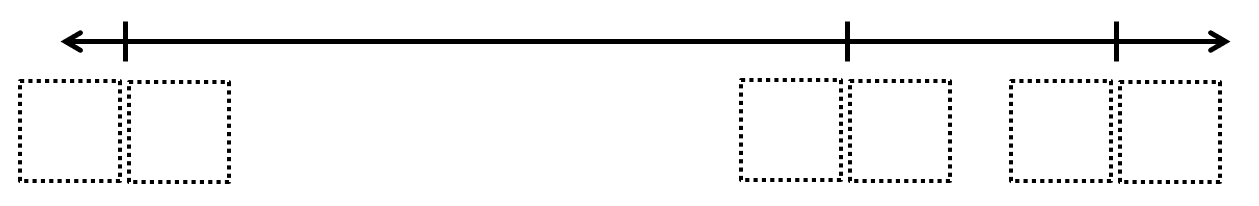# Open Number Line

Directions: Use the digits 1 to 9, at most one time each, place a digit in each box on the number line to make the number line true. NOTE: number line not drawn to scale### Hint

Example start in the middle with 39, what numbers could make this a true number line.

There are many answers including 17, 39, 42 and 35, 86, 92

Source: Amanda Dey

### Check Also

Directions: Use the digits 1-9, at most once, to complete the equation. What is the …

1.24,39,156

2.24, 39, 78

3.24 39 57

4.•The directions said to use the digits 1 through 9 at most one time each, so we can’t use 0 even once, much less three times.

5.I posed this problem on an assignment for my math for preservice elementary teachers class, and I was surprised at how many missed it. Some would re-use a digit, some used 0, and some wrote a two-digit number in each box.

6.7.Robert Kaplinsky

I’m wondering about whether we should just pay attention to the placement or whether we should also be paying attention to the spacing on the number line. There should not be infinite answers if spacing matters.